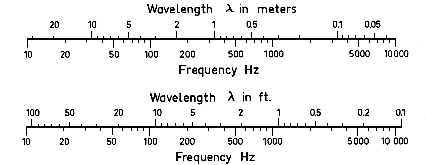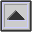WAVELENGTH

For any PERIODIC wave the wavelength is the distance from a given point in the wave to the corresponding point in the next cycle of the wave, frequently represented by the Greek letter lambda (l). It may also be thought of as the distance the sound travels in one CYCLE or PERIOD.

The velocity v of sound (see SPEED OF SOUND) in any medium equals the frequency f times the wavelength (v = f.l). Similarly if the velocity and frequency are known, the wavelength may be determined by dividing the velocity by the frequency (l = v / f). See chart below.

Some examples of the wavelengths of various frequencies are:

 lowest audible C 16.4 Hz 69 feet lowest C on piano 32.7 Hz 34 feet 6 inches middle C on piano 261 Hz 4 feet 3 inches violin A string 440 Hz 2 feet 6 inches C four octaves above middle C 4,186 Hz 3.25 inches highest audible tone 20,000 Hz 0.68 inchesScales for wavelength to frequency conversion for the speed of sound in air of 343 m/sec.

home